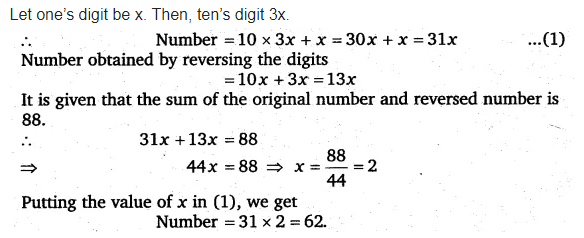# One of the two digits of a two digit number is three times the other digit

One of the two digits of a two digit number is three times the other digit. If you interchange the digits of this two-digit number and add the resulting number to the original number, you get 88. What is the original number ?